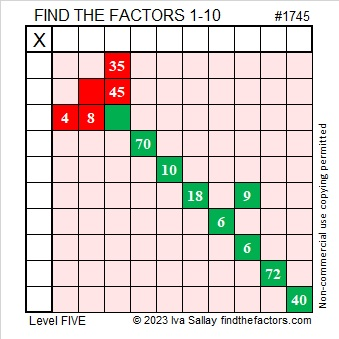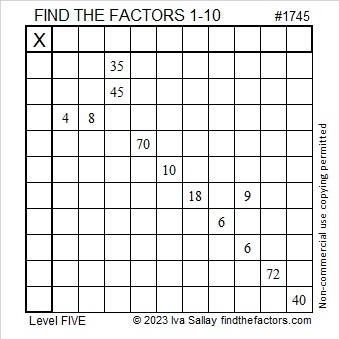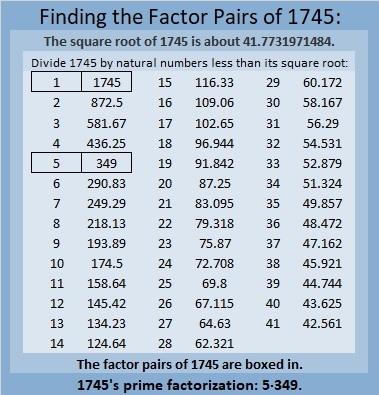# 1745 How Thorny Is This Rose?

Contents

### Today’s Puzzle:

How thorny is this Valentine’s rose? That depends on if you use logic to solve it! It is a Level 5 puzzle, so some of the clues are meant to be tricky. Nevertheless, you can solve it! Just place all the numbers 1 to 10 in both the first column and the top row so that the given clues are the products of the numbers you write. There is only one solution.Here’s the same puzzle minus the color:### Factors of 1745:

• 1745 is a composite number.
• Prime factorization: 1745 = 5 × 349.
• 1745 has no exponents greater than 1 in its prime factorization, so √1745 cannot be simplified.
• The exponents in the prime factorization are 1 and 1. Adding one to each exponent and multiplying we get (1 + 1)(1 + 1) = 2 × 2 = 4. Therefore 1745 has exactly 4 factors.
• The factors of 1745 are outlined with their factor pair partners in the graphic below.### More About the Number 1745:

1745 is the difference of two squares in two different ways:
873² – 872² = 1745, and
177² – 172² = 1745.

1745 is also the sum of two squares in two different ways:
41² + 8² = 1745, and
31² + 28² = 1745.

1745 is the hypotenuse of FOUR Pythagorean triples:
177-1736-1745, calculated from 31² – 28², 2(31)(28), 31² + 28²,
656-1617-1745, calculated from 2(41)(8), 41² – 8², 41² + 8²,
900-1495-1745, which is 5 times (180-299-349)
1047-1396-1745, which is (3-4-5) times 349.

This site uses Akismet to reduce spam. Learn how your comment data is processed.